# How to Calculate and Solve for Intensity of Induced Magnetization | Magnetic Field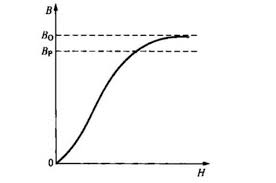The image above represents intensity induced magnetization.

To compute for intensity induced magnetization, three essential parameters are needed and these parameters are Magnetic Moment (M), Length (L) and Cross-Sectional Area (A).

The formula for calculating intensity induced magnetization:

J = M / LA

Where:

J = Intensity of Induced Magnetization
M = Magnetic Moment
L = Length
A = Cross-Sectional Area

Let’s solve an example;
Find the intensity induced magnetization when the magnetic moment is 20, the length is 16 and the cross-sectional area is 25.

This implies that;

M = Magnetic Moment = 20
L = Length = 16
A = Cross-Sectional Area = 25

J = M / LA
J = 20 / (16)(25)
J = 20 / 400
J = 0.05

Therefore, the intensity induced magnetization is 0.05.

Calculating the Magnetic Moment when the Intensity Induced Magnetization, the Length and the Cross-Sectional Area is Given.

M = J x LA

Where;

M = Magnetic Moment
J = Intensity Induced Magnetization
L = Length
A = Cross-Sectional Area

Let’s solve an example;
Find the Magnetic Moment when the intensity induced magnetization is 20, the length is 12 and the cross-sectional area is 5.

This implies that;

J = Intensity Induced Magnetization = 20
L = Length = 12
A = Cross-Sectional Area = 5

M = J x LA
M = 20 x (12)(5)
M = 20 x 60
M = 1200

Therefore, the magnetic moment is 1200.

Calculating the Length when the Intensity Induced Magnetization, the Magnetic Moment and the Cross-Sectional Area is Given.

L = M / J x A

Where;

L = Length
J = Intensity Induced Magnetization
M = Magnetic Moment
A = Cross-Sectional Area

Let’s solve an example;
Find the length when the intensity induced magnetization is 15, the magnetic moment is 42 and the cross-sectional area is 3.

This implies that;

J = Intensity Induced Magnetization = 15
M = Magnetic Moment = 42
A = Cross-Sectional Area = 3

L = M / J x A
L = 42 / 15 x 3
L = 42 / 45
L = 0.93

Therefore, the length is 0.93.

Calculating the Cross-Sectional Area when the Intensity Induced Magnetization, the Magnetic Moment and the Length is Given.

A = M / J x L

Where;

A = Cross-Sectional Area
J = Intensity Induced Magnetization
M = Magnetic Moment
L = Length

Let’s solve an example;
Find the Cross-Sectional Area when the Intensity Induced Magnetization is 30, the length is 9 and the magnetic moment is 60.

This implies that;

J = Intensity Induced Magnetization = 30
M = Magnetic Moment = 60
L = Length = 9

A = M / J x L
A = 60 / 30 x 9
A = 60 / 270
A = 0.22

Therefore, the intensity induced magnetization is 0.22

Nickzom Calculator – The Calculator Encyclopedia is capable of calculating the intensity induced magnetization.

To get the answer and workings of the intensity induced magnetization using the Nickzom Calculator – The Calculator Encyclopedia. First, you need to obtain the app.

You can get this app via any of these means:

To get access to the professional version via web, you need to register and subscribe for NGN 2,000 per annum to have utter access to all functionalities.
You can also try the demo version via https://www.nickzom.org/calculator

Apple (Paid) – https://itunes.apple.com/us/app/nickzom-calculator/id1331162702?mt=8
Once, you have obtained the calculator encyclopedia app, proceed to the Calculator Map, then click on Geology under Add-on.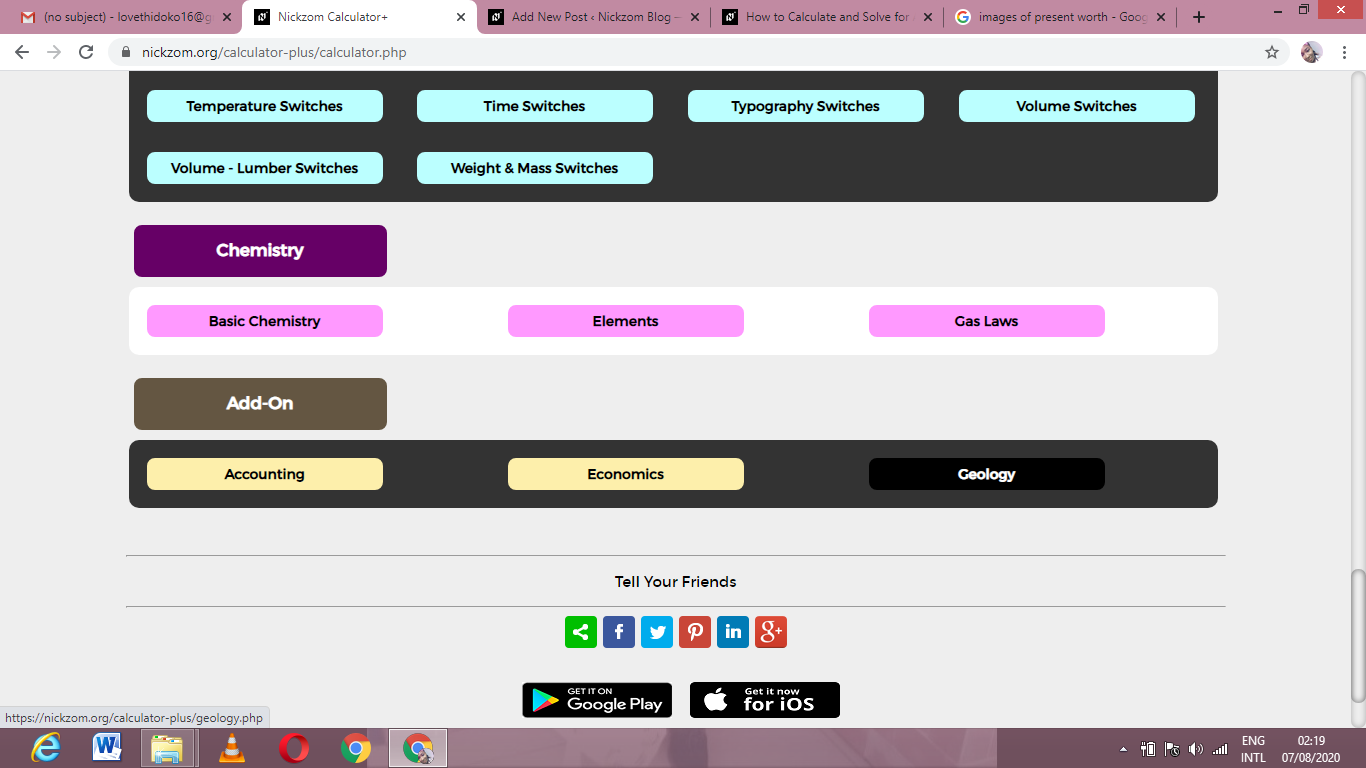Now, Click on Magnetic Field under GeologyNow, Click on Intensity Induced Magnetization under Magnetic Field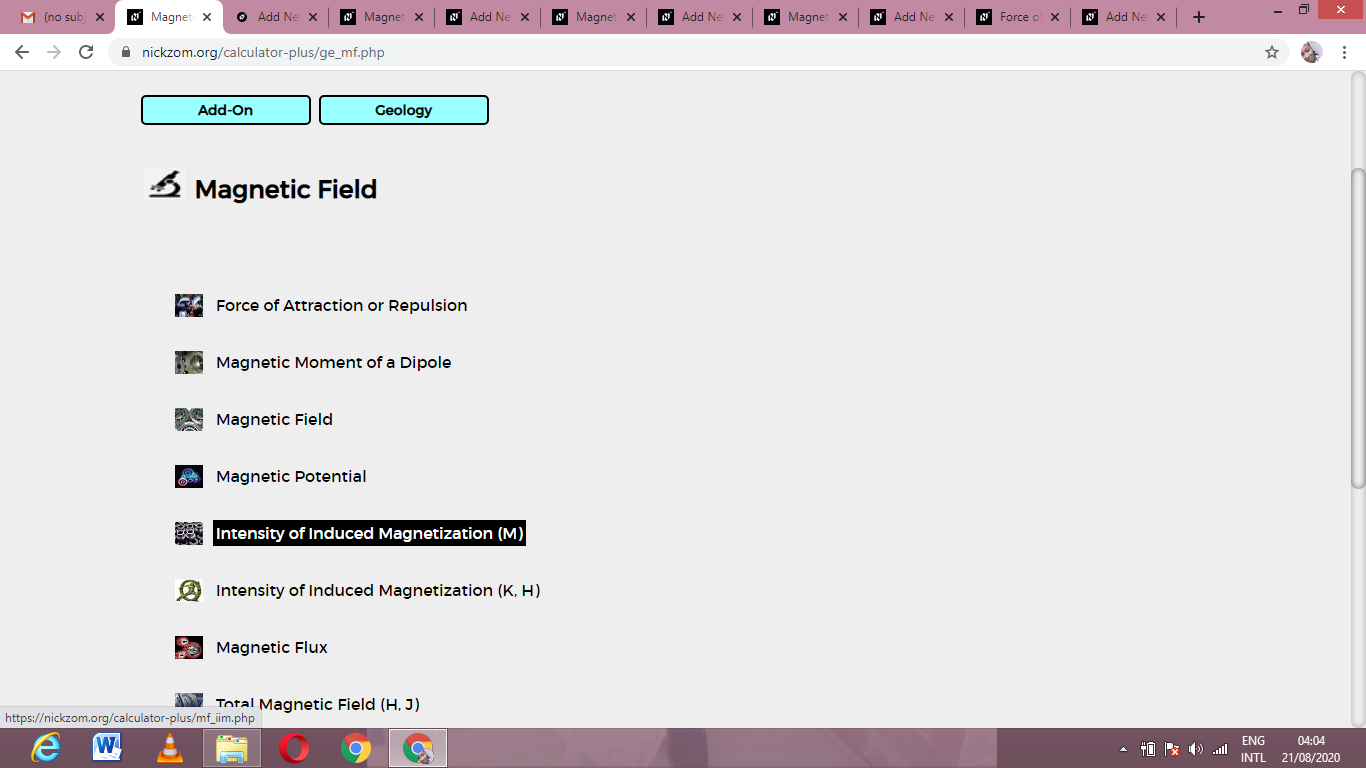The screenshot below displays the page or activity to enter your values, to get the answer for the intensity induced magnetization according to the respective parameters which are the Magnetic Moment (M), Length (L) and Cross-Sectional Area (A).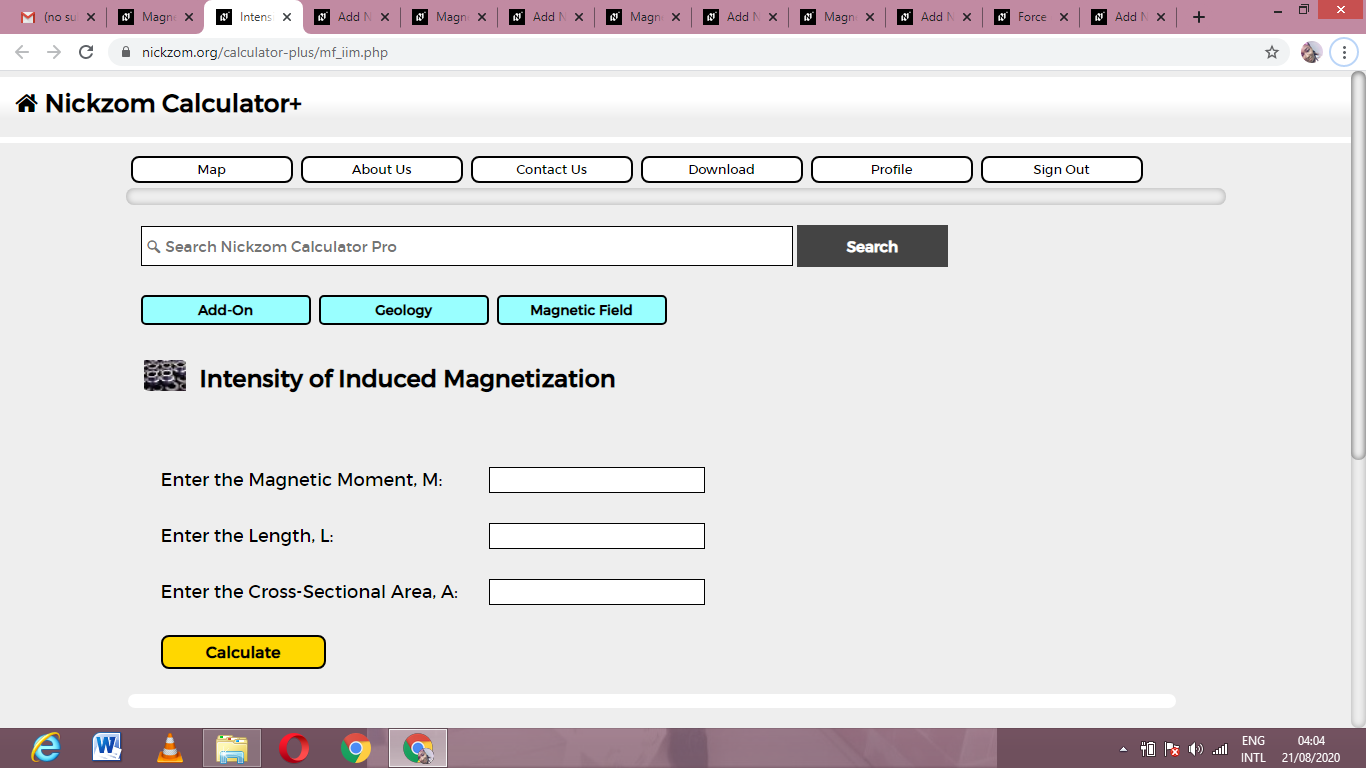Now, enter the values appropriately and accordingly for the parameters as required by the Magnetic Moment (M) is 20, Length (L) is 16 and Cross-Sectional Area (A) is 25.Finally, Click on Calculate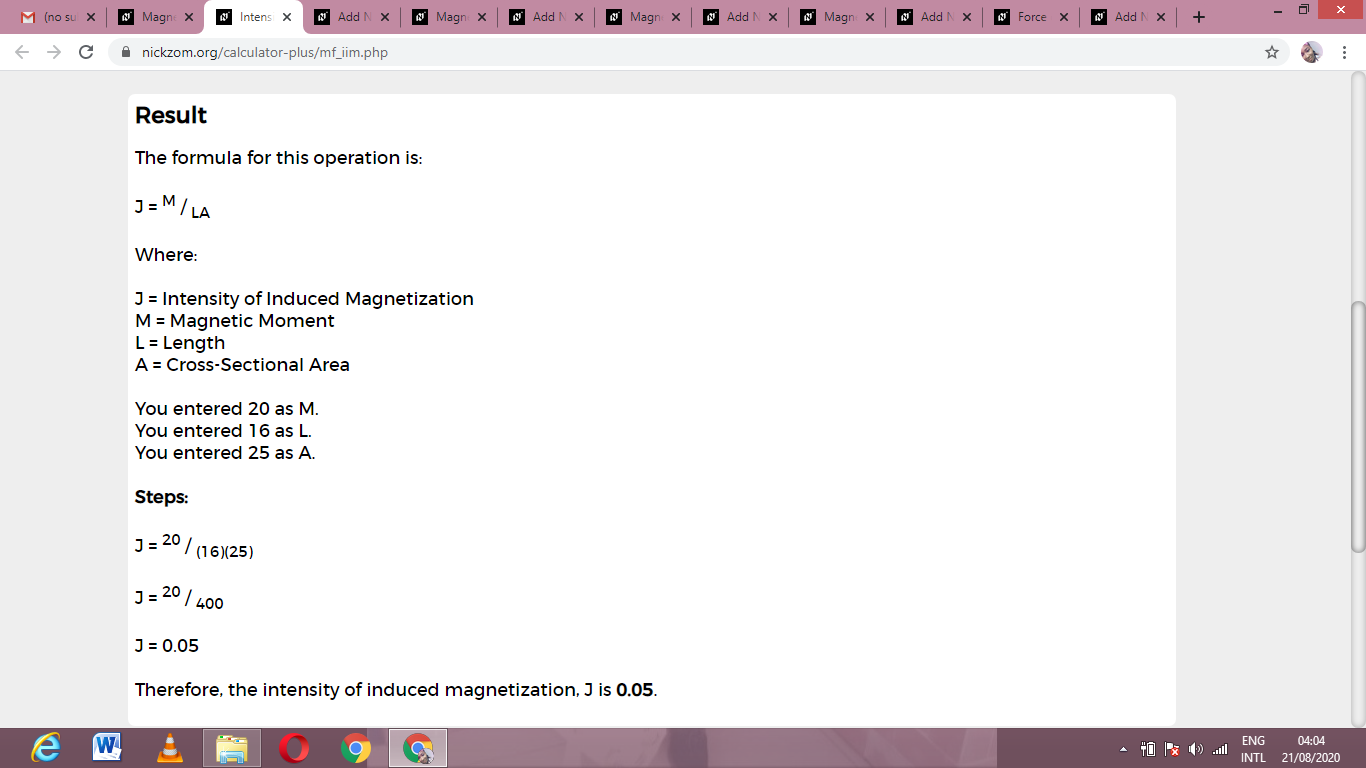As you can see from the screenshot above, Nickzom Calculator– The Calculator Encyclopedia solves for the intensity induced magnetization and presents the formula, workings and steps too.